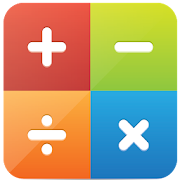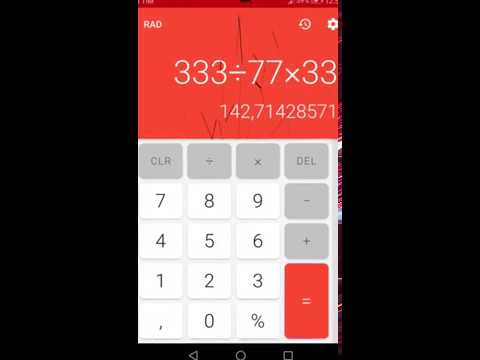# Calculator very fast & simpleEveryone
205Free calculator! Combine the functions of a basic calculator and a scientific calculator. Color your days by customizing the calculator with the colors that you like the most. You can customize the colors without paying any extra charge.
Active scientific calculator to perform more complex calculations, or use the memory buttons like those of the original calculator.
You can do many mathematical operations with the calculator. It comprises: addition, subtraction, division, multiplication, percentage, to the cube elevation, elevation nth power, power of 2, cube root, power to the base 10 and multiple of 3 exponent, n-th root, power of E , the power of 10, square, square root, natural logarithm, common logarithm, binary logarithm, sine, cosine, tangent, arc sine, cosine, hyperbolic sine, hyperbolic cosine, hyperbolic tangent, arc tangent, inverse hyperbolic sine, hyperbolic arc tangent, mutual, random number and factorial.
For example, Calculator is perfect in situations such as calculating taxes, homework study for school or university, calculations at work, add up the receipts, calculate the tip in restaurants and calculate the discount while you shop.
Features:
- Simple and elegant design
- Easy to use with large buttons
- Customize your calculator with the colors that you like the most
- Option to activate / deactivate the vibrator
- Option to enable / disable memory buttons
- View your calculations with commas
- Basic calculation buttons
- Buttons of advanced mathematical and scientific functions
Collapse

Review Policy
4.3
205 total
5
4
3
2
1

## What's New

bug fixes
Collapse

Updated
July 2, 2019
Size
Varies with device
Installs
100,000+
Current Version
Varies with device
Requires Android
Varies with device
Content Rating
Everyone
Permissions
Offered By
Together - App4you
Developer